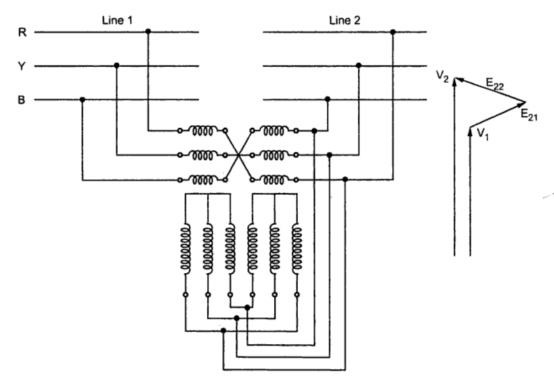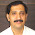Three Phase Induction Regulators

The polyphase (especially three phase) induction regulators are similarly in construction to that of three phase slip ring induction motors with wound primary and secondary windings. The primary is connected across the lines while the secondary is connected on the load side in series with the lines. Here compensating coils are not required. Also slip rings are not used as there is limited movement of rotor. The flexible connections are used on rotor which acts as primary winding. The connection diagram is as shown in the Fig. 1.Fig. 1
The electric and magnetic circuits are almost similar to that of slip ring induction motor. The output voltage can be varied by changing the angular position of the rotor which results in phase shift but no change in magnitude of secondary induced emfs. The currents in the primary windings produce rotating magnetic field revolving at synchronous speed around the air gap. The emfs induced in the secondary winding are constant irrespective of angular positions of stator and rotor.
The phase of secondary voltage changes with respect to that of primary with the movement of rotor.
Let      Vbe the supply voltage
Vbe the load side voltage
Ebe the induced emf in the regulator
The induced emf in the induction regulator can be uniformly changed from V1+Vto V1-V by changing the rotor position through 180 electrical degrees. This is shown in the Fig. 2.Fig. 2
The above phasor diagram for the three phases is shown in the Fig. 3.Fig. 3
The limiting voltage regulation which can be obtained from the regulator is as shown in the Fig. 4.Fig. 4
The rotor can be turned either in the direction of or against the direction of rotating magnetic field produced by primary. The output current I2 is displaced from output voltage V by phase angle or power factor angle Φ2. Io is no load current while I2' is current carried by primary winding. If  N1 and N are number of turns on primary and secondary windings then,
I2'/I2 = N2/N1 = E2/V1
The total supply current I1 remains same even after rotating the rotor in the direction or against the dierction of rotating magnetic field as shown in the Fig. 4. The current taken by primary winding changes as per the rotor movement. The phasor diagram for all the three phases can be drawn in the similar manner.
The supply voltage and output voltage are in phase when stator and rotor axes are same and are displaced from each other by 180o. For all intermediate positions, angle δ exist between these two voltages (viz  V and V2 ). Such phase displacement may give rise to large circulating currents in certain cases which can be avoided by using either a twin regulator or a bank of single phase units.
A twin regulator consists of two coupled three phase regulators each of rating equal to half the total boost KVA required. The secondary windings are connected in series and rotors of each are always moved in opposite directions. This arrangement opposes the torques produced due to induction motor action and neutralizes to each other considerably which would otherwise required to be taken up by the control devices in a single unit of three phase induction regulator.
This torque is dependent on the rotor position, the power factor of the load and the load current. If power factor is unity, the torque is maximum for maximum buck or boost position (i.e. stator and rotor axes separated by either 180o or 0o) while the torque is zero for rotor displacement of 90o at unity power factor.
The expression for torque is given by,
T = k V1 I2' cos (θ ± Φ2)
When two induction regulators are coupled to form a twin regulator, the effective torque is proportional to cos(θ + Φ2)-cos(θ - Φ2). Thus for unity power factor load, the torque always cancel each other compeletely.
The twin regulator is diagramatically represented in the Fig.5.Fig. 5 Twin type three phase induction regulator
Whenever line voltage is 11 kV or more, direct connection of line with primary winding is not possible. In such case suitable transformer is used to supply the regulator primary and inject secondary emf in main circuit.
The rating of regulator is product of its full load current, voltage boost and number of phases. Consider a 3 ph, 11000 V system with full load line current of 100 A. To get ±10% variation, regulator rating is,
Rating of regulator in KVA = √3x 11 x 10+3 x (10/100) x 100 x 10-3 = 190.52
The regulators are normally immersed in oil tanks provided with cooling tubes closely resembling that of transformer.

1.